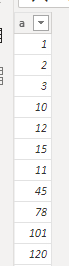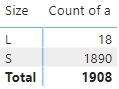cancel
Showing results for
Did you mean:Frequent Visitor

## Is there an equivalent concept of the "dimension calculated field" of QuickSight in PowerBI?

Hi, there

I am just moving from QuickSight to PowerBI. Everything looks good except for one concept. In QuickSight, there are two types of calculated fields:

• One is the "calculated measure field", which could be used for the "value" of "Matrix" visual
• The other is the "calculated dimension field", which could be used for "row" or "column" of the "Matrix" visual (it also means you can use a calculated field for grouping)

I believe "measure" in PowerBI equals the "calculated measure field" of QuickSight. But what is the equivalent "calculated dimension field" in PowerBI?

Hao

1 ACCEPTED SOLUTIONSuper User

@Hao , Got we can have calculated column based on parameter. what you need do I create measure which gives that value

``Size = IF(Max(Table1[a]) < Threshold[Parameter Value], "S", "L")``

Have a table with values S and L , Say Bucket

You need row level id column in the table, if required add index column in the power bi

new Measure
Sumx(filter(Values(Table[Index]), [size] = max(bucket[Value])), [a])

or see if this can work

Sumx(filter(Table, [size] = max(bucket[Value])), [a])

refer

Dynamic segmentation -Measure to Dimension conversion: https://youtu.be/gzY40NWJpWQ

4 REPLIES 4Super UserFrequent Visitor

Hi @amitchandak,

Thanks for your quick reply! I look at this new feature. It improved but still has a gap with the "calculated dimension field". The key difference is:

- PowerBI field parameter requires either column (one time of one-to-one transformation) or measure (dynamic N to one aggregation)

- QuickSight "calculated dimension field" is a dynamic one-to-one transformation

In summary, QuickSight "calculated dimension field" is "PowerBI field parameter" + "dynamic one-to-one transformation".

Step1: I have a table "Table1" with a single numeric column "a":Step 2: I have a what-if parameter "Threshold" to allow the user to choose a threshold.

Step 3: I want to add a measure "Size" to "Table1" like

``Size = IF(Table1[a] < Threshold[Parameter Value], "S", "L")``

Step 4: Finally, I am trying to use "Size" as a "field parameter" to form a Matrix visual like belowUnfortunately, I didn't figure out a way to do that because of step 3. "Size" is a measure that requires an "aggregation operator" (e.g., SUM, AVERAGE). QuickSight "calculated dimension field" has no such restriction.

HaoSuper User

@Hao , Got we can have calculated column based on parameter. what you need do I create measure which gives that value

``Size = IF(Max(Table1[a]) < Threshold[Parameter Value], "S", "L")``

Have a table with values S and L , Say Bucket

You need row level id column in the table, if required add index column in the power bi

new Measure
Sumx(filter(Values(Table[Index]), [size] = max(bucket[Value])), [a])

or see if this can work

Sumx(filter(Table, [size] = max(bucket[Value])), [a])

refer

Dynamic segmentation -Measure to Dimension conversion: https://youtu.be/gzY40NWJpWQFrequent Visitor

@amitchandak, you pointed me in the right direction! Thank you so much!

My final formula looks like this:

``COUNTX(FILTER(Table1, countrows(filter(Bucket, Table1[Size] == Bucket[Size])) > 0), [a])``

But the complexity of the computation of my version is O(M*N), in which N is the size of "Table1", and M is the size of "Bucket". When M or N is huge, the refresh of the page is extremely slow.

Your version looks more optimized (much faster than mine):

``countx(filter(Table1, [size] = max(Bucket[Size])), [a])``

However, it doesn't return the correct result for me ("max" causes "S" to be used in comparison forever); what is your original intention of "max"? I hope to learn it for optimization.Hao